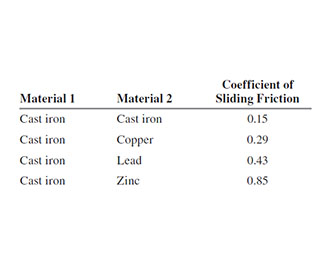# Problem: A long ramp made of cast iron is sloped at a constant angle kg = 52.0m/s above the horizontal. Small blocks, each with mass 0.42 m kg but made of different materials, are released from rest at a vertical height h above the bottom of the ramp. In each case the coefficient of static friction is small enough that the blocks start to slide down the ramp as soon as they are released. You are asked to find h so that each block will have a speed of 4.00 m m/s when it reaches the bottom of the ramp. You are given these coefficients of sliding (kinetic) friction for different pairs of materials .Use work and energy considerations to find the required value of h if the block is made from cast iron.Use work and energy considerations to find the required value of h if the block is made from copper.Use work and energy considerations to find the required value of h if the block is made from zinc.What is the required value of h for the copper block if its mass is doubled to 0.84 kg?For a given block, if heta is increased while h is kept the same, does the speed v of the block at the bottom of the ramp increase, decrease, or stay the same?

###### FREE Expert Solution
79% (479 ratings)
###### Problem Details

A long ramp made of cast iron is sloped at a constant angle kg = 52.0m/s above the horizontal. Small blocks, each with mass 0.42 but made of different materials, are released from rest at a vertical height h above the bottom of the ramp. In each case the coefficient of static friction is small enough that the blocks start to slide down the ramp as soon as they are released. You are asked to find h so that each block will have a speed of 4.00 when it reaches the bottom of the ramp. You are given these coefficients of sliding (kinetic) friction for different pairs of materials.

Use work and energy considerations to find the required value of h if the block is made from cast iron.

Use work and energy considerations to find the required value of h if the block is made from copper.

Use work and energy considerations to find the required value of h if the block is made from zinc.

What is the required value of h for the copper block if its mass is doubled to 0.84 kg?

For a given block, if is increased while is kept the same, does the speed of the block at the bottom of the ramp increase, decrease, or stay the same?

Frequently Asked Questions

What scientific concept do you need to know in order to solve this problem?

Our tutors have indicated that to solve this problem you will need to apply the Conservative Forces & Inclined Planes concept. You can view video lessons to learn Conservative Forces & Inclined Planes. Or if you need more Conservative Forces & Inclined Planes practice, you can also practice Conservative Forces & Inclined Planes practice problems.